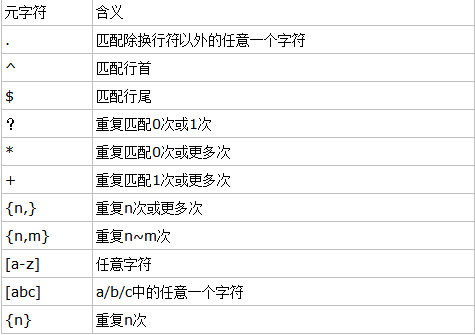# Java及python正则表达式详解

更新时间：2017年12月27日 15:12:32   投稿：mdxy-dxy我要评论\  :将下一个字符标记为一个特殊字符、或一个原义字符、或一个 向后引用、或一个八进制转义符。例如，'n' 匹配字符 "n"。'\n' 匹配一个换行符。序列 '\\' 匹配 "\" 而 "\(" 则匹配 "("。

‘[a-zA-Z0-9]'：匹配字母或数字
‘[^abc]'：匹配除abc之外的字母
‘p(ython|erl)'匹配Python和perl
‘(pattern)*'匹配0次或多次
‘(pattern)+'匹配1次或多次
‘(pattern){m,n}'匹配m_n次
‘(a|b)*c'匹配0-n次a或b后面紧跟c
‘^[a-zA-Z]{1}([a-zA-Z0-9\._]){4,19}\$'匹配20个字符以字母开始
‘^(\w){6,20}\$'匹配6-20个单词字符
‘^\d{1,3}\.\d{1,3}\.\d{1,3}\.\d{1,3}\$'匹配IP
‘^[a-zA-Z]+\$'检查字符中只包含英文字母
‘\w+@(\w+\.)\w+\$'匹配邮箱
‘[\u4e00-\u9fa5]'匹配汉字
‘^\d{18|\d{15}\$'匹配身份证
‘\d{4}-\d{1,2}-\d{1,2}'匹配时间
‘^(?=.*[a-z])(?=.*[A-Z])(?=.*\d)(?=.*[,._]).{8,}\$)'判断是否为强密码
‘(.)\\1+'匹配任意字符的一次或多次出现

re模块常用方法介绍：

compile(pattern[,flags])创建模式对象
search(pattern,string[,flags])在整个字符中寻找模式，返回match对象或者None
match(pattern,string[,flags])从字符串的开始处于匹配模式，返回匹配对象
findall(pattern,string[,flags])：列出匹配模式中的所有匹配项
split(pattern,string[,maxsplit=0])根据匹配模式分割字符串
sub(pat,repl,string[,count=0])将字符串中所有pat匹配项用repl替换
escape(string)将字符中的所有特殊正则表达式字符转义

match，search，findall区别

match在字符串开头或指定位置进行搜索，模式必须出现在开头或指定位置；
search方法在整个字符串或指定位置进行搜索；
findall在字符串中查找所有符合正则表达式的字符串并以列表返回。

```>>> import re
>>> m = re.match(r'(\w+) (\w+)','Isaac Newton,physicist')
>>> m.group(0)
'Isaac Newton'
>>> m.group(1)
'Isaac'
>>> m.group(2)
'Newton'
>>> m.group(1,2)
('Isaac', 'Newton')
>>>m=re.match(r'(?P<first_name>\w+)(?P<last_name>\w+)','Malcolm Reynolds')
>>> m.group('first_name')
'Malcolm'
>>> m.group('last_name')
'Reynolds'
>>> m.groups()
('Malcolm', 'Reynolds')
>>> m.groupdict()
{'first_name': 'Malcolm', 'last_name': 'Reynolds'}```

```>>> import re
>>> exampleString = '''There should be one--and preferably only one--obvious way to do it.
Although that way may not be obvious at first unless you're Dutch.
Now is better than never.
Although never never is often better than right now.'''
>>> pattern = re.compile(r'(?<=\w\s)never(?=\s\w)')
>>> matchResult = pattern.search(exampleString)
>>> matchResult.span()
(171, 176)
>>> pattern = re.compile(r'(?<=\w\s)never')
>>> matchResult = pattern.search(exampleString)
>>> matchResult.span()
(154, 159)
>>> pattern = re.compile(r'(?:is\s)better(\sthan)')
>>> matchResult = pattern.search(exampleString)
>>> matchResult.span()
(139, 153)
>>> matchResult.group(0)
'is better than'
>>> matchResult.group(1)
' than'
>>> pattern = re.compile(r'\b(?i)n\w+\b')
>>> index = 0
>>> while True:
matchResult = pattern.search(exampleString,index)
if not matchResult:
break
print(matchResult.group(0),':',matchResult.span(0))
index = matchResult.end(0)

not : (90, 93)
Now : (135, 138)
never : (154, 159)
never : (171, 176)
never : (177, 182)
now : (210, 213)
>>> pattern = re.compile(r'(?<!not\s)be\b')
>>> index = 0
>>> while True:
matchResult = pattern.search(exampleString,index)
if not matchResult:
break
print(matchResult.group(0),':',matchResult.span(0))
index = matchResult.end(0)

be : (13, 15)
>>> exampleString[13:20]
'be one-'
>>> pattern = re.compile(r'(\b\w*(?P<f>\w+)(?P=f)\w*\b)')
>>> index = 0
>>> while True:
matchResult = pattern.search(exampleString,index)
if not matchResult:
break
print(matchResult.group(0),':',matchResult.group(2))
index = matchResult.end(0)+1

unless : s
better : t
better : t
>>> s = 'aabc abbcd abccd abbcd abcdd'
>>> p = re.compile(r'(\b\w*(?P<f>\w+)(?P=f)\w*\b)')
>>> p.findall(s)
[('aabc', 'a'), ('abbcd', 'b'), ('abccd', 'c'), ('abbcd', 'b'), ('abcdd', 'd')] ```